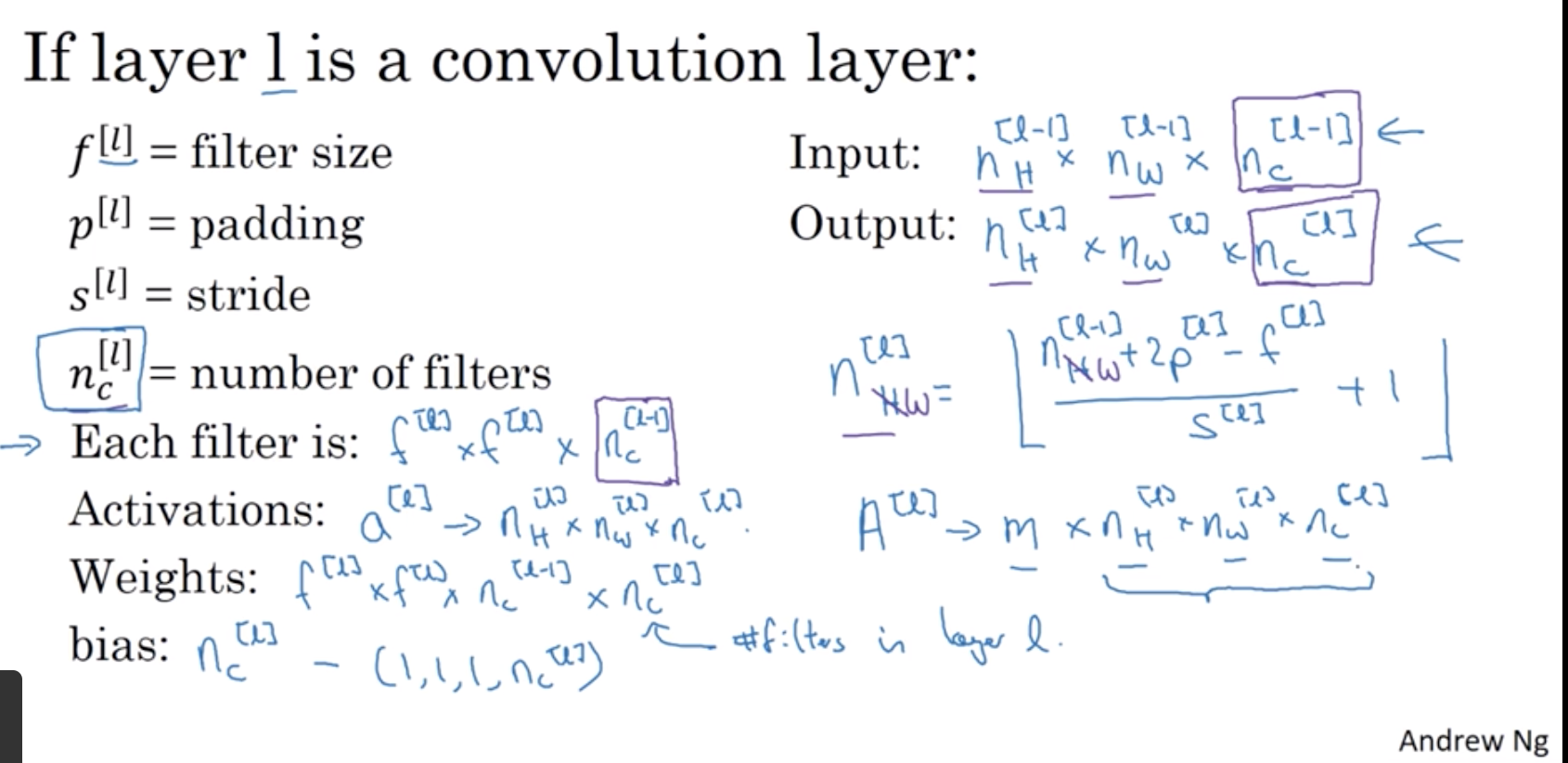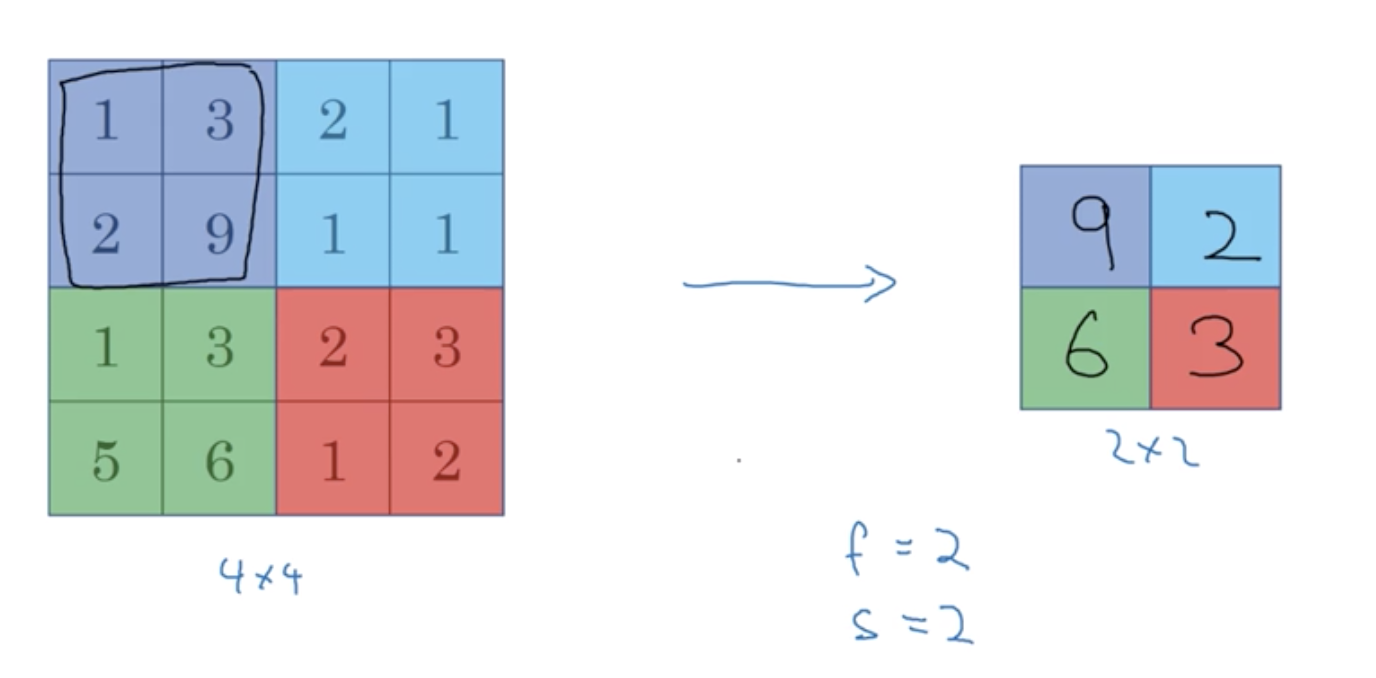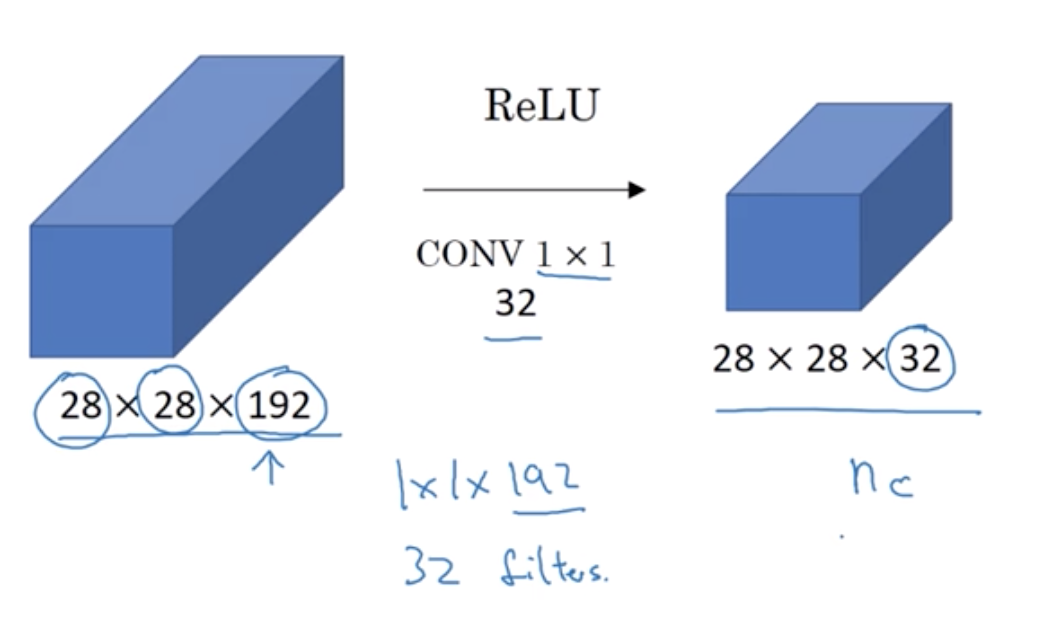RY 's Blog

YOLO - From Configuration File to Convolutional Layers

2019-05-08

Let’s firstly see how Darknet construct a neural network. See at detector.c test_detector function, it construct a network by parsing the xxx.cfg file and xxx.weights file. In my case, they are yolo3-tiny.cfg and yolo3-tiny.weights

I am trying to understand Darknet source code that implements YOLO algorithm. First, I run the detector.

Parse the argumenets

In main function, it goes to function test_detector according to the first argumentdetect.

Here is the architecture of neural network defined by yolov3-tiny.cfg

Now, let’s see how Darknet construct a nerual network. See detector.c test_detector function, it construct a network by parsing the xxx.cfg file and xxx.weights file. In my case, they are yolo3-tiny.cfg and yolo3-tiny.weights

Parse the configuration file

Sections in the file

The code to parse the yolo.cfg file is here:

for exmaple:

[net]

In section ‘[net]’, batch=1 is a option stored in kvp(option_list.c line 70) structure. Its key is batch, value is 1. Then this kvp object will be inserted into a node list (see it at option_list.c line76 & list.c line 40).
After parsing the yolo3-tiny.cfg file, We will get a section list; its size is 25. Because there are 25 \’[\’ tags in yolo3-tiny.cfg

In parse_network_cfg function, it parses the [net] section to get the params for the whole network.

[convolutional]

Then parse the different sections.

For the first [convolutional] section in the yolo3-tiny.cfg as follow, the darknet will construct a convolutional_layer using thess params (see function parse_convolutional in parse.c and function make_convolutional_layer in convolutional_layer.c)

In this layer, there are 16 filters; the size of each filter is 3X3Xnum_channel; what is num_channel? well, the number of channels in a filter must match the number of channels in input volume, so here num_channel is equal to 3. The stride value for filters is 1, padding value is 1.

Let’s see how darknet calculate the output size of convolutional_layer by the input size(l.h) and filter params (l.size, l.pad, l.stride). There is a formula that shows how size of input volume relates to the one of output volume

As for yolo3-tiny.cfg, for this first convolutional_layer, its input size is 416 x 416 and channel is 3. So its ouput height is (416+2x1 - 3)/1 + 1 = 416, its output width is 416 too. What about its output channel? It equals to the number of filters (16).

So its output volume size is 416 X 416 X 16.For a beginner, I strongly recommend these courses: Strided Convolutions - Foundations of Convolutional Neural Networks | Coursera and One Layer of a Convolutional Network - Foundations of Convolutional Neural Networks | Coursera

Now, we have 16 filters that are 3X3X3 in this layer, how many parameters does this layer have? Each filter is a 3X3X3 volume, so it’s 27 numbers tp be learned, and then plus the bias, so that was the b parameters. it’s 28 parameters. There are 16 filters so that would be 448 parameters to be learned in this layer.

Activation

In this convolution layer, it choose leaky ReLU as activation function. The function is defined as follow where α is a small constant.

$$f(x)=\begin{cases} αx,\quad x\leq 0 \\ x,\quad x>0 \end{cases}$$

Still, I recommend this course for a beginner. Activation functions - Shallow neural networks | Coursera

There are forward_activation_layer and backward_activation_layer in Darknet. Both of them handle batch inputs.

For forward activation layer, leaky_activate is to computes f(x)

For backward activation layer, leaky_gradient returns the slop of the function

[maxpool]

Maxpool layer is used to reduce the size of representation to speed up computation as well as to make some of the features it detects a bit more robust. Look at the tiny-yolo3.cfg

This [maxpool] sections comes after the [convolutional] section. Its input size(416 x 416 x 16) equal to the output size of the former layer (416 x 416 x 16). The filter size is 2 x 2, stride is 2. Each time, the filter would move 2 steps, for a 4x4x1 input volume, its output is 2x2x1 volume.So in this layer, its ouput width equals to (int)((416+ 1 - 2)/2 + 1), 208. And the number of its output channels equals to the number of input channels. Now, we know its output volume size is 208 X 208 X 16. There is no parameter to be learned.

input volume size:

$$n_H . n_W . n_c$$

$n_c$ : the number of channels

output volume size:

$$(\frac{n_H + padding-f}{stride} + 1) . (\frac{n_W + padding-f}{stride} +1) . n_c$$

$f$: the width or height of a filter

Why does 1 x 1 convolution do?

For example, in this picture, the number of input volume channels ,192, has gotten too big, we can shrink it to a 28x28x32 dimension volume using 32 filters that are 1x1x192. So this is a way to shrink the number of channels .In YOLO, it implements fully connected layer by two convolutional layer.

Tags: YOLO